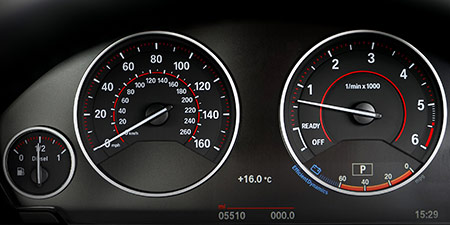# Speed of sound to Meters per second (m/s)

Thema Units of speed ﹣ Converter Speed of sound to Meters per second

Here you can convert the Speed unit Speed of sound into the unit Meters per second and vice versa you can convert Meters per second into Speed of sound. By clicking the "Swap units" icon, you will always obtain the desired conversion in the calculation result. With the following calculator you can also calculate any other Speed unit.

## Info about "Speed of sound"

Definition: If an object travels at the speed of sound in air at 20 degrees Celsius for one second, it travels a distance of 343 meters per second. Actually, it is equivalent to 1.234,8 km/h.

The speed of sound is often illustrated by an example of a thunderstorm: if lightning strikes within 3 seconds of the thunder sound, then the thunderstorm is about 1 kilometer away. Another example, in a 100-meter sprint, the starting shot is fired directly next to the starting blocks. Imagine, if the start shot was fired at the finish line, it would take about 0.3 seconds of time to hear the shot in the start blocks.

## Info about "Meters per second"

Meters per second is a basic unit for speed in the International System of Units (SI) and other metric systems. This is because both meter and second are the international base units for distance and time. Definition of "meters per second": An object moving at 1 "m/s" per second will cover a distance of one meter.

In our daily work, speed is commonly measured in km/h. The following rule is an easy way to remember when converting m/s into km/h: &qquot; multiply by four and minus 10 %". For example, standard walking speeds in meters per second are about 1 m/s for a walker, 10.44 m/s is the world record for running 100 meters, and the speed of sound is 343 meters per second.

As mentioned above, both meter and second are the international base units for distance and time. All other speed units in the metric system are derived based on meters per second (e.g. km/h, mm/s).

## Formula for the conversion of Speed of sound to Meters per second (m/s) and vice versa

The calculation from Speed of sound to Meters per second shall be made using the following conversion formula:

Conversion formula Speed of sound to Meters per second

Determine the number of Meters per second from Speed of sound

Speed of sound × 343

## Formula for the conversion of Meters per second (m/s) to Speed of sound

The calculation from Meters per second to Speed of sound shall be made using the following conversion formula:

Conversion formula Meters per second to Speed of sound

Determine the number of Speed of sound from Meters per second

Meters per second × 0.0029154518950437

## Overview table : How many Speed of sound are in Meters per second ?

Speed of sound ⇒ Meters per second m/s
0.01   are  3.43000 m/s
0.02   are  6.86000 m/s
0.03   are  10.29000 m/s
0.04   are  13.72000 m/s
0.05   are  17.15000 m/s
0.06   are  20.58000 m/s
0.07   are  24.01000 m/s
0.08   are  27.44000 m/s
0.09   are  30.87000 m/s
0.10   are  34.30000 m/s
0.20   are  68.60000 m/s
0.30   are  102.90000 m/s
0.40   are  137.20000 m/s
0.50   are  171.50000 m/s
0.60   are  205.80000 m/s
0.70   are  240.10000 m/s
0.80   are  274.40000 m/s
0.90   are  308.70000 m/s
1   corresponds to  343 m/s
2   are  686 m/s
3   are  1 029 m/s
4   are  1 372 m/s
5   are  1 715 m/s
6   are  2 058 m/s
7   are  2 401 m/s
8   are  2 744 m/s
9   are  3 087 m/s
10   are  3 430 m/s
20   are  6 860 m/s
30   are  10 290 m/s
40   are  13 720 m/s
50   are  17 150 m/s
60   are  20 580 m/s
70   are  24 010 m/s
80   are  27 440 m/s
90   are  30 870 m/s
100   are  34 300 m/s
200   are  68 600 m/s
300   are  102 900 m/s
400   are  137 200 m/s
500   are  171 500 m/s
600   are  205 800 m/s
700   are  240 100 m/s
800   are  274 400 m/s
900   are  308 700 m/s
1 000   are  343 000 m/s

## Overview table : How many Meters per second are in Speed of sound ?

Meters per second m/s ⇒ Speed of sound
0.01 m/s  are  0.00002
0.02 m/s  are  0.00005
0.03 m/s  are  0.00008
0.04 m/s  are  0.00011
0.05 m/s  are  0.00014
0.06 m/s  are  0.00017
0.07 m/s  are  0.00020
0.08 m/s  are  0.00023
0.09 m/s  are  0.00026
0.10 m/s  are  0.00029
0.20 m/s  are  0.00058
0.30 m/s  are  0.00087
0.40 m/s  are  0.00116
0.50 m/s  are  0.00145
0.60 m/s  are  0.00174
0.70 m/s  are  0.00204
0.80 m/s  are  0.00233
0.90 m/s  are  0.00262
1 m/s  corresponds to  0.00291
2 m/s  are  0.00583
3 m/s  are  0.00874
4 m/s  are  0.01166
5 m/s  are  0.01457
6 m/s  are  0.01749
7 m/s  are  0.02040
8 m/s  are  0.02332
9 m/s  are  0.02623
10 m/s  are  0.02915
20 m/s  are  0.05830
30 m/s  are  0.08746
40 m/s  are  0.11661
50 m/s  are  0.14577
60 m/s  are  0.17492
70 m/s  are  0.20408
80 m/s  are  0.23323
90 m/s  are  0.26239
100 m/s  are  0.29154
200 m/s  are  0.58309
300 m/s  are  0.87463
400 m/s  are  1.16618
500 m/s  are  1.45772
600 m/s  are  1.74927
700 m/s  are  2.04081
800 m/s  are  2.33236
900 m/s  are  2.62390
1 000 m/s  are  2.91545

## Source information

As source for the information in the "Units of speed" category, we have used in particular:

## Last update on May 3, 2022

The pages of the "Units of speed" category were last editorially reviewed by Stefan Banse on May 3, 2022. They all correspond to the current status.

### Previous changes on October 9, 2021

• November 9, 2020: Publication of the Speed converter
• Editorial revision of all texts in this category